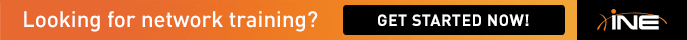dynagen crashes everytime

Hello Friends,

i am trying to run INE .net file with config in dynagen in WINDOWS XP since three days but still crashing everytime on 9the device.

what to do?

here is my .net file config for dynagen, please suggest me?

##############################################################################################

#

# Internetwork Expert Routing & Switching topology Version 5.0 mappings for dynamips/GNS3 on Mac OS X

#

#

# Dynagen: http://dyna-gen.sourceforge.net/

# GNS3: http://www.gns3.net

# Hacki's Forum: http://hacki.at/7200emu/index.php

# Internetwork Expert's Forum: http://IEOC.com

#

##############################################################################################

autostart = False

[localhost:7200]

workingdir = G:Documents and SettingsOwnerDesktopVakel

[]

ram = 128

ghostios = True

[[ROUTER R1]]

model = 3725

console = 2001

idlemax = 100

s0/0 = FR0 1

s0/1 = R3 s1/2

f0/0 = SW1 f1/1

[[ROUTER R2]]

model = 3725

console = 2002

idlemax = 100

s0/0 = FR0 2

s0/1 = R3 s1/3

f0/0 = SW2 f1/2

[[ROUTER R3]]

model = 3725

console = 2003

idlemax = 100

f0/0 = SW1 f1/3

f0/1 = SW3 f1/3

slot1 = NM-4T

s1/0 = FR0 3

s1/1 = FR0 4

s1/2 = R1 s0/1

s1/3 = R2 s0/1

[[ROUTER R4]]

model = 3725

console = 2004

idlemax = 100

s0/0 = FR0 5

s0/1 = R5 s0/1

f0/0 = SW2 f1/4

f0/1 = SW4 f1/4

[[ROUTER R5]]

model = 3725

console = 2005

idlemax = 100

s0/0 = FR0 6

s0/1 = R4 s0/1

f0/0 = SW1 f1/5

f0/1 = SW3 f1/5

[[ROUTER R6]]

model = 3725

console = 2006

idlemax = 100

s0/0 = BB1 s0/0

f0/0 = SW2 f1/6

f0/1 = SW4 f1/6

[[ROUTER SW1]]

model = 3725

console = 2007

idlemax = 100

slot1 = NM-16ESW

f1/1 = R1 f0/0

f1/3 = R3 f0/0

f1/5 = R5 f0/0

f1/7 = SW2 f1/7

f1/8 = SW2 f1/8

f1/9 = SW2 f1/9

f1/10 = SW3 f1/7

f1/11 = SW3 f1/8

f1/12 = SW3 f1/9

f1/13 = SW4 f1/7

f1/14 = SW4 f1/8

f1/15 = SW4 f1/9

[[ROUTER SW2]]

model = 3725

console = 2008

idlemax = 100

slot1 = NM-16ESW

f1/0 = BB2 f0/0

f1/2 = R2 f0/0

f1/4 = R4 f0/0

f1/6 = R6 f0/0

f1/7 = SW1 f1/7

f1/8 = SW1 f1/8

f1/9 = SW1 f1/9

f1/10 = SW3 f1/10

f1/11 = SW3 f1/11

f1/12 = SW3 f1/12

f1/13 = SW4 f1/10

f1/14 = SW4 f1/11

f1/15 = SW4 f1/12

[[ROUTER SW3]]

model = 3725

console = 2009

idlemax = 100

slot1 = NM-16ESW

f1/0 = BB3 f0/0

f1/3 = R3 f0/1

f1/5 = R5 f0/1

f1/7 = SW1 f1/10

f1/8 = SW1 f1/11

f1/9 = SW1 f1/12

f1/10 = SW2 f1/10

f1/11 = SW2 f1/11

f1/12 = SW2 f1/12

f1/13 = SW4 f1/13

f1/14 = SW4 f1/14

f1/15 = SW4 f1/15

[[ROUTER SW4]]

model = 3725

console = 2010

idlemax = 100

slot1 = NM-16ESW

f1/4 = R4 f0/1

f1/6 = R6 f0/1

f1/7 = SW1 f1/13

f1/8 = SW1 f1/14

f1/9 = SW1 f1/15

f1/10 = SW2 f1/13

f1/11 = SW2 f1/14

f1/12 = SW2 f1/15

f1/13 = SW3 f1/13

f1/14 = SW3 f1/14

f1/15 = SW3 f1/15

[[FRSW FR0]]

1:102 = 2:201

1:103 = 3:301

1:104 = 5:401

1:105 = 6:501

1:113 = 4:311

2:201 = 1:102

2:203 = 3:302

2:204 = 5:402

2:205 = 6:502

2:213 = 4:312

3:301 = 1:103

3:302 = 2:203

3:304 = 5:403

3:305 = 6:503

4:311 = 1:113

4:312 = 2:213

4:314 = 5:413

4:315 = 6:513

5:401 = 1:104

5:402 = 2:204

5:403 = 3:304

5:405 = 6:504

5:413 = 4:314

6:501 = 1:105

6:502 = 2:205

6:503 = 3:305

6:504 = 5:405

6:513 = 4:315

[[ROUTER BB1]]

model = 3725

idlemax = 100

console = 2011

#cnfg = G:Documents and SettingsOwnerDesktopVakelCCIE WorbooksINE Initial configBB1.cfg

s0/0 = R6 s0/0

s0/1 = BB3 s0/0

[[ROUTER BB2]]

model = 3725

console = 2012

#cnfg = G:Documents and SettingsOwnerDesktopVakelCCIE WorbooksINE Initial configBB2.cfg

f0/0 = SW2 f1/0

idlemax = 100

[[ROUTER BB3]]

model = 3725

idlemax = 100

console = 2013

#cnfg = G:Documents and SettingsOwnerDesktopVakelCCIE WorbooksINE Initial configBB3.cfg

s0/0 = BB1 s0/1

f0/0 = SW3 f1/0

[GNS3-DATA]

configs = initial.configs

m11 = 1.0

m22 = 1.0

• [localhost:7200]

workingdir = G:Documents and SettingsOwnerDesktopVakel

Those lines open a hypervisor. Hypervisors are the engines of the routers. Generate three or four of them to get load off it. Here is my .net file where you can see how I made it.

http://pastebin.com/rmeR1iBK

Tell me if it worked!

Regards!

Markus

• Thank you so much for the post.